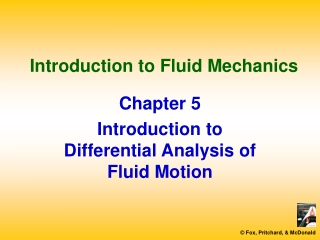# Introduction to Fluid Mechanics - PowerPoint PPT PresentationDownload PresentationIntroduction to Fluid Mechanics

Introduction to Fluid Mechanics
Download Presentation## Introduction to Fluid Mechanics

- - - - - - - - - - - - - - - - - - - - - - - - - - - E N D - - - - - - - - - - - - - - - - - - - - - - - - - - -
##### Presentation Transcript

1. Introduction to Fluid Mechanics Chapter 5 Introduction to Differential Analysis of Fluid Motion

2. Main Topics • Conservation of Mass • Stream Function for Two-Dimensional Incompressible Flow • Motion of a Fluid Particle (Kinematics) • Momentum Equation

3. Conservation of Mass • Basic Law for a System

4. Conservation of Mass • Rectangular Coordinate System

5. Conservation of Mass • Rectangular Coordinate System

6. Conservation of Mass • Rectangular Coordinate System “Continuity Equation”

7. Conservation of Mass • Rectangular Coordinate System “Del” Operator

8. Conservation of Mass • Rectangular Coordinate System

9. Conservation of Mass • Rectangular Coordinate System Incompressible Fluid: Steady Flow:

10. Conservation of Mass • Cylindrical Coordinate System

11. Conservation of Mass • Cylindrical Coordinate System

12. Conservation of Mass • Cylindrical Coordinate System “Del” Operator

13. Conservation of Mass • Cylindrical Coordinate System

14. Conservation of Mass • Cylindrical Coordinate System Incompressible Fluid: Steady Flow:

15. Stream Function forTwo-DimensionalIncompressible Flow • Two-Dimensional Flow • Stream Function y

16. Stream Function forTwo-DimensionalIncompressible Flow • Cylindrical Coordinates • Stream Function y(r,q)

17. Motion of a Fluid Particle (Kinematics) • Fluid Translation: Acceleration of aFluid Particle in a Velocity Field • Fluid Rotation • Fluid Deformation • Angular Deformation • Linear Deformation

18. Motion of a Fluid Particle (Kinematics)

19. Motion of a Fluid Particle (Kinematics) • Fluid Translation: Acceleration of aFluid Particle in a Velocity Field

20. Motion of a Fluid Particle (Kinematics) • Fluid Translation: Acceleration of aFluid Particle in a Velocity Field

21. Motion of a Fluid Particle (Kinematics) • Fluid Translation: Acceleration of aFluid Particle in a Velocity Field

22. Motion of a Fluid Particle (Kinematics) • Fluid Translation: Acceleration of aFluid Particle in a Velocity Field (Cylindrical)

23. Motion of a Fluid Particle (Kinematics) • Fluid Rotation

24. Motion of a Fluid Particle (Kinematics) • Fluid Rotation

25. Motion of a Fluid Particle (Kinematics) • Fluid Rotation

26. Motion of a Fluid Particle (Kinematics) • Fluid Deformation: • Angular Deformation

27. Motion of a Fluid Particle (Kinematics) • Fluid Deformation: • Angular Deformation

28. Motion of a Fluid Particle (Kinematics) • Fluid Deformation: • Linear Deformation

29. Momentum Equation • Newton’s Second Law

30. Momentum Equation • Forces Acting on a Fluid Particle

31. Momentum Equation • Forces Acting on a Fluid Particle

32. Momentum Equation • Differential Momentum Equation

33. Momentum Equation • Newtonian Fluid: Navier-Stokes Equations

34. Momentum Equation • Special Case: Euler’s Equation

35. Computational Fluid Dynamics • Some Applications

36. Computational Fluid Dynamics • Discretization